# 1 Introduction

• 某些领域专家的偏好：一些领域的专家有一些自己喜欢用的模型。
• 可用的数据很少：避免过拟合，提供insight，使用预训练的模型进行迁移也很可取。
• 资源有限：当电力内存受限时，简单模型更可取。

# 2 General Framework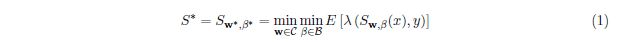## 2.1 Algorithm Description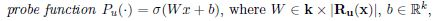$\sigma(\cdot)$是softmax，$k$是类别数，在数据集$D_N$上进行训练。$P_u(R_u(x))[y]\in[0,1]$就是标签$y$的confidence score。

ProWeight算法在Algorithm 1中展示步骤（5）计算权重可以使用AUG算法（Algorithm 2），或是将confidence scores作为输入的正则化神经网络，正则项为$R(w)=(\frac{1}{m}\Sigma^m_{i=1}w_i-1)^2$，并且神经网络在batch上训练，正则项要计算所有的样本。然后使用weights在$D_S$上训练简单样本就好了。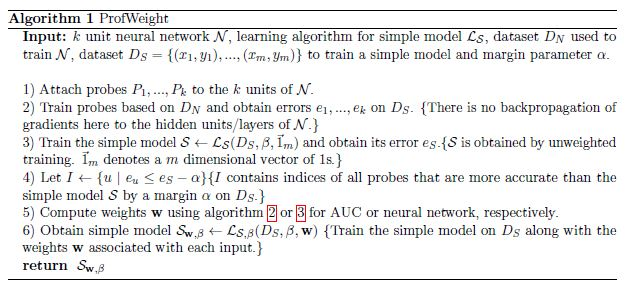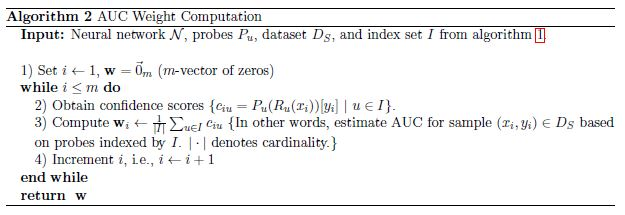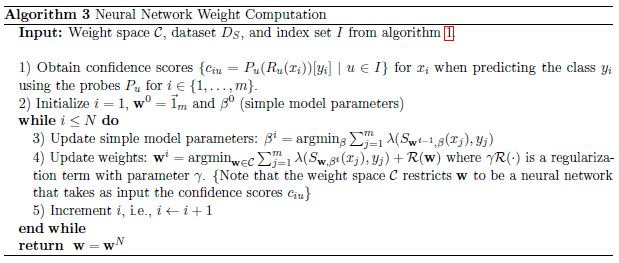## 2.2 Theoretical Justification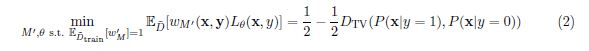# 3 Experiments

## 3.1 CIFAR-10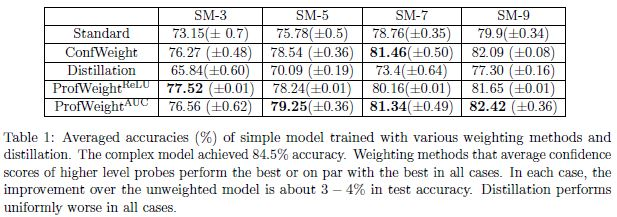## 3.2 Manufacturing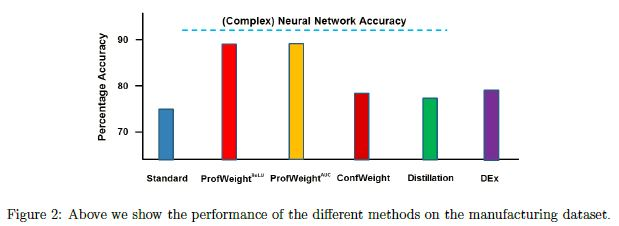# 4 Related Work and Discussion

©️2019 CSDN 皮肤主题: 大白 设计师: CSDN官方博客